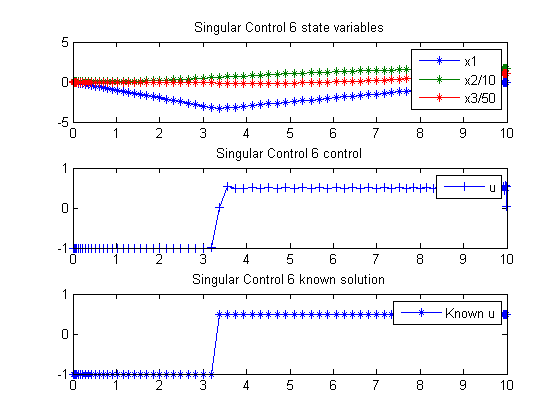106  Singular Control 6

Viscocity Solutions of Hamilton-Jacobi Equations and Optimal Control Problems. Alberto Bressan, S.I.S.S.A, Trieste, Italy.

A singular control example.

106.1  Problem Description

Find u over t in [0; 10 ] to maximize:

 J = x3(tf)

subject to:

 dx1 dt
= u
 dx2 dt
= −x1
 dx2 dt
= x2x12
 x(t0) = [0  0  0]
 |u| <= 1

Reference: 

106.2  Problem setup

toms t
t_F = 10;
p = tomPhase('p', t, 0, t_F, 80);
setPhase(p);

tomStates x1 x2 x3
tomControls u

x = [x1; x2; x3];

% Initial guess
x0 = {icollocate({x1 == 0, x2 == 0, x3 == 0})
collocate(u==0)};

% Box constraints
cbox = {-1 <= collocate(u) <= 1};

% Boundary constraints
cbnd = initial(x == [0;0;0]);

% ODEs and path constraints
ceq = collocate({dot(x1) == u; dot(x2) == -x(1)
dot(x3) == x(2)-x(1).^2});

% Objective
objective = -final(x(3));

106.3  Solve the problem

options = struct;
options.name = 'Singular Control 6';
solution = ezsolve(objective, {cbox, cbnd, ceq}, x0, options);

% Extract optimal states and controls from solution
t  = collocate(subs(t,solution));
u  = collocate(subs(u,solution));
x1 = collocate(subs(x1,solution));
x2 = collocate(subs(x2,solution));
x3 = collocate(subs(x3,solution));
Problem type appears to be: lpcon
Starting numeric solver
===== * * * =================================================================== * * *
TOMLAB - Tomlab Optimization Inc. Development license  999001. Valid to 2011-02-05
=====================================================================================
Problem: ---  1: Singular Control 6             f_k     -55.555568442322624000
sum(|constr|)      0.000000011396340832
f(x_k) + sum(|constr|)    -55.555568430926286000
f(x_0)      0.000000000000000000

Solver: snopt.  EXIT=0.  INFORM=1.
SNOPT 7.2-5 NLP code
Optimality conditions satisfied

FuncEv    1 ConstrEv   58 ConJacEv   58 Iter   49 MinorIter  678
CPU time: 1.750000 sec. Elapsed time: 1.781000 sec.

106.4  Plot result

subplot(3,1,1)
plot(t,x1,'*-',t,x2/10,'*-',t,x3/50,'*-');
legend('x1','x2/10','x3/50');
title('Singular Control 6 state variables');

subplot(3,1,2)
plot(t,u,'+-');
legend('u');
title('Singular Control 6 control');

subplot(3,1,3)
plot(t,-1*(t<t_F/3)+1/2*(t>=t_F/3),'*-');
legend('Known u');
title('Singular Control 6 known solution');